# The Crossing Number of the Complete Bipartite Graph

 Importance: High ✭✭✭
 Author(s): Turan, Paul
 Subject: Graph Theory » Topological Graph Theory » » Crossing numbers
 Keywords: complete bipartite graph crossing number
 Posted by: Robert Samal on: May 11th, 2007

The crossing number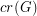ofis the minimum number of crossings in all drawings ofin the plane.

Conjecture(This discussion appears as [M].)

A drawing of a graphin the plane has the vertices represented by distinct points and the edges represented by polygonal lines joining their endpoints such that:

\item no edge contains a vertex other than its endpoints, \item no two adjacent edges share a point other than their common endpoint, \item two nonadjacent edges share at most one point at which they cross transversally, and \item no three edges cross at the same point.

This problem is also known as Turan's Brickyard Problem (since it was formulated by Turan when he was working at a brickyard - the edges of the drawing would correspond to train tracks connecting different shipping depots, and fewer crossings would mean smaller chance for collision of little trains and smaller chance for their derailing).

This conjectured value for the crossing number ofcan be realized by the following drawing. Place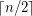vertices on the positive-axis and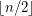vertices on the negative-axis. Similarly, place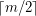andalong the positive and negative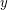-axis. Now connect each pair of vertices on different axes with straight line segments.

## Bibliography

[G] R. Guy, The decline and fall of Zarankiewicz's theorem, in Proof Techniques in Graph Theory (F. Harary Ed.), Academic Press, New York (1969) 63-69.

[K] D. Kleitman, The crossing number of K_{5,n} , J. Combin. Theory 9 (1970) 315-32

[M] B. Mohar, Problem of the Month

* indicates original appearance(s) of problem.